# Area Under The Curve - Calculus

In Calculus, we have seen definite integral as a limit of a sum and we know how to evaluate it using the fundamental theorem of calculus. In the upcoming discussion, we will see an easier way of finding the area bounded by any curve and x-axis between given coordinates. To determine the area under the curve we follow the below method.

## How to Determine the Area Under the Curve?

Let us assume the curve y=f(x) and its ordinates at the x-axis be x=a and x=b. Now, we need to evaluate the area bounded by the given curve and the ordinates given by x=a and x=b.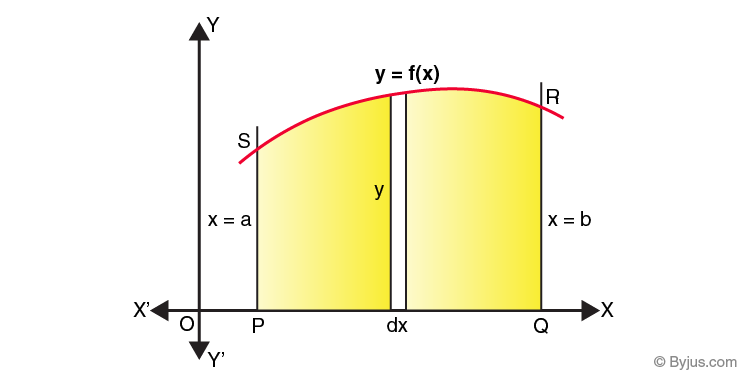The area under the curve can be assumed to be made up of many vertical, extremely thin strips. Let us take a random strip of height y and width dx as shown in the figure given above whose area is given by dA.

The area dA of the strip can be given as y dx. Also, we know that any point of the curve, y is represented as f(x). This area of the strip is called an elementary area. This strip is located somewhere between x=a and x=b, between the x-axis and the curve. Now, if we need to find the total area bounded by the curve and the x-axis, between x=a and x=b, then it can be considered to be made of an infinite number of such strips, starting from x=a to x=b. In other words, adding the elementary areas between the thin strips in the region PQRSP will give the total area.

Mathematically, it can be represented as:

$$\begin{array}{l} A = \int\limits_{a}^b dA = \int\limits_{a}^b ydx = \int\limits_{a}^b f(x)dx\end{array}$$

Using the same logic, if we want to calculate the area under the curve x=g(y), y-axis between the lines y=c and y=d, it will be given by: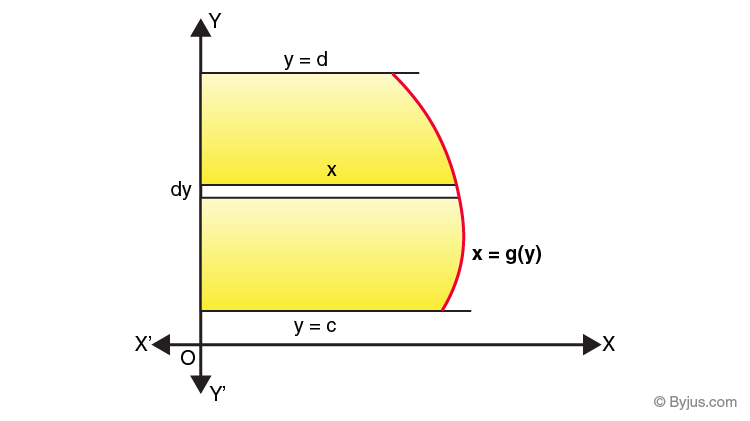$$\begin{array}{l} A = \int\limits_{c}^d xdy = \int\limits_{c}^d g(y)dy\end{array}$$

In this case, we need to consider horizontal strips as shown in the figure above.

Also, note that if the curve lies below the x-axis, i.e. f(x) <0 then following the same steps, you will get the area under the curve and x-axis between x=a and x=b as a negative value. In such cases, take the absolute value of the area, without the sign, i.e.|

$$\begin{array}{l}\int\limits_{a}^b f(x)dx|\end{array}$$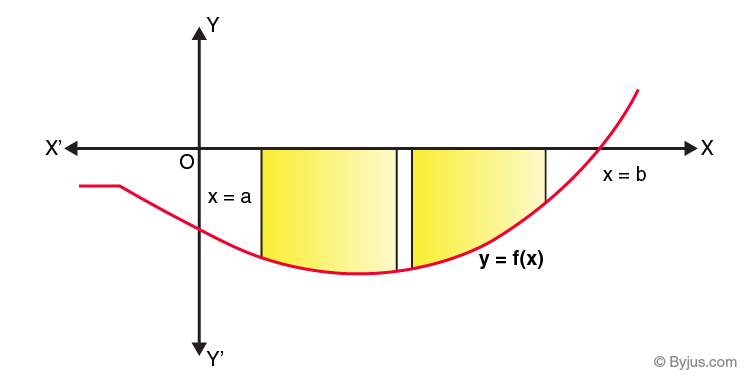Another possibility is that, when some portion of the curve may lie above the x-axis and some portion below the x-axis, as shown in the figure,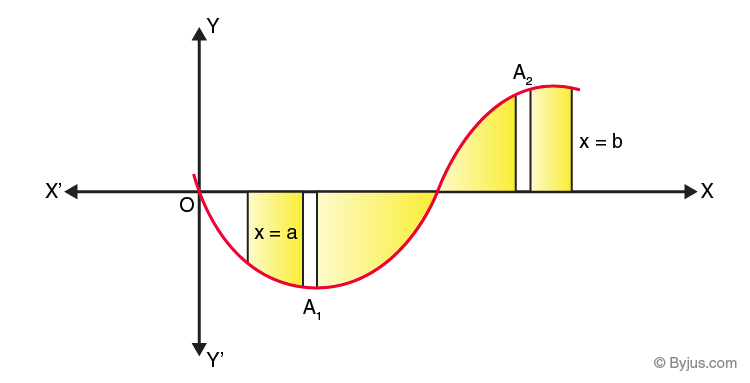Here A1<0 and A2>0. Hence, this is the combination of the first and second case. Hence, the total area will be given as |A1|+A2

### Area Under the Curve Example

Let us consider an example, to understand the concept in a better way.

We need to find the total area enclosed by the circle x2+y2=1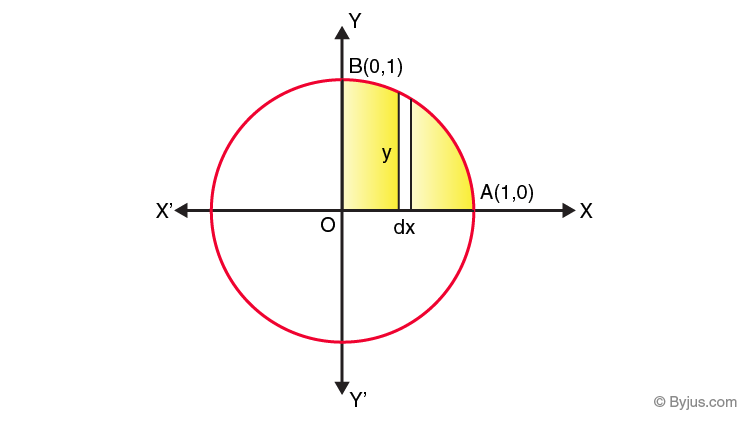Area enclosed by the whole circle = 4 x area enclosed OABO

=4

$$\begin{array}{l}\int\limits_{0}^1 ydx \end{array}$$
(considering vertical strips)

$$\begin{array}{l} =4 \int\limits_{0}^1 \sqrt{{1}-{x}^{2}}\end{array}$$

On integrating, we get,

$$\begin{array}{l}=4 [\frac{x}{2}\sqrt{{1}-{x}^{2}}+ \frac{1}{2} {sin}^{-1}x{]}_{0}^{1}\end{array}$$

= 4 × 1/2 x π/2

= π

So the required area is π square units.